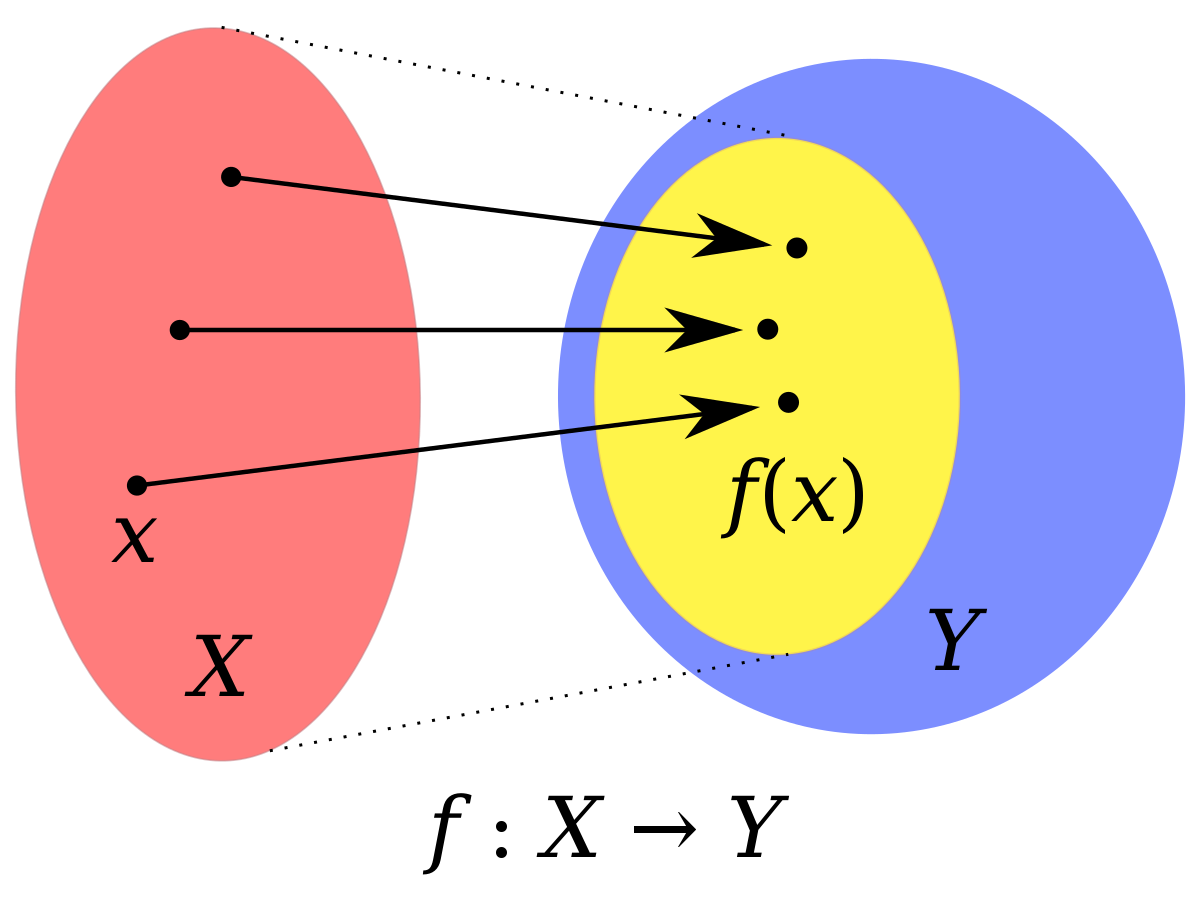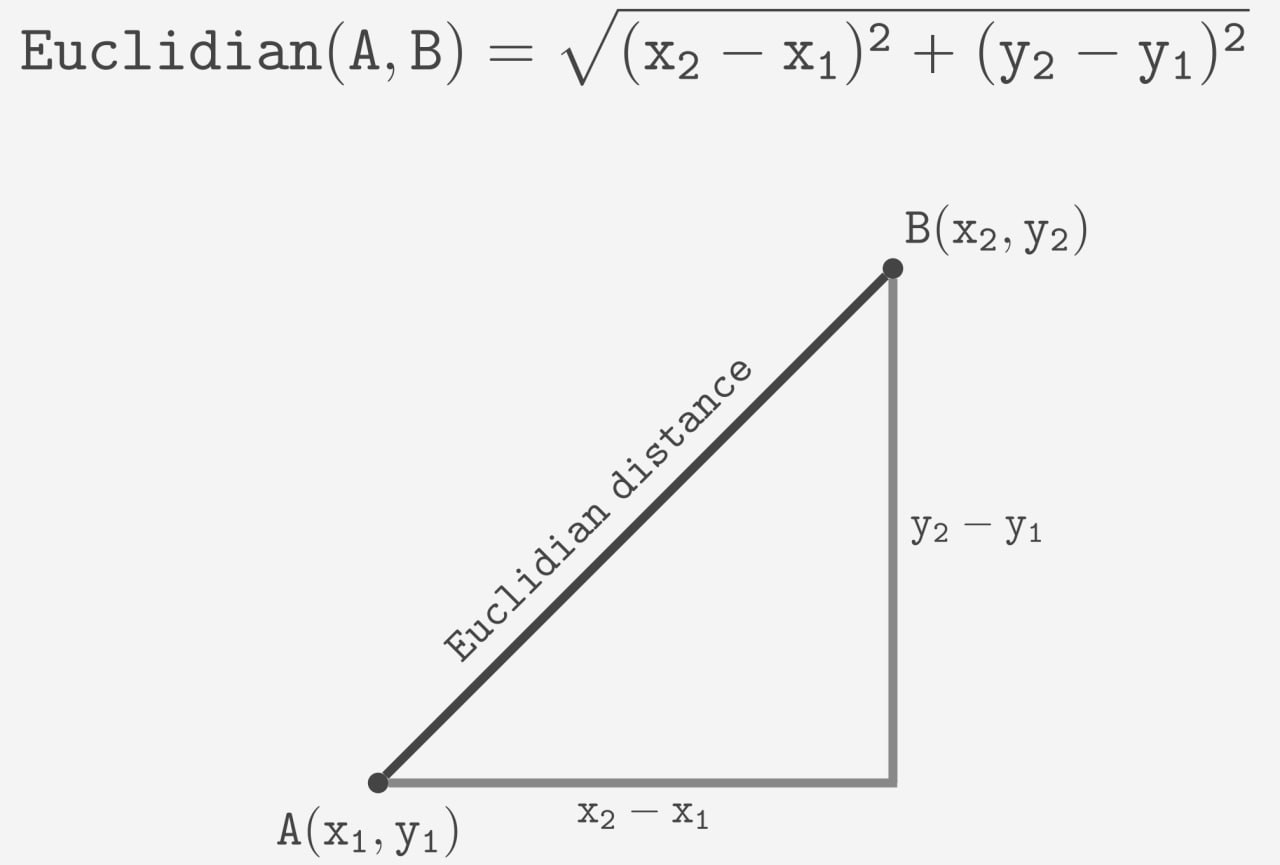Course Content

Mathematics for Data Analysis and Modeling

## Theory

In mathematics, a function is a fundamental concept that describes a relationship between two sets of objects, known as the domain and the codomain. A function assigns an output value to each input value from the domain.

Formally, a function can be defined as follows:

Given two sets, `X` and `Y`, a function `f` from `X` to `Y` is a rule that assigns each element in `X` (domain), called the input, to an element in `Y` (codomain), called the output. It is denoted as:

`f: X → Y`It is important to note that functional dependencies are always unambiguous: only one value from codomain Y can be assigned to a value from domain X. But simultaneously, several values from X can correspond to one value from Y.

## Examples of functions

Let's look at the example to understand it. Assume that you have two points at the plane and want to calculate the Euclidean distance between these points. To do it, we use the distance function:We have a domain consisting of coordinates of points and a codomain representing distances. The Euclidean function assigns a distance to each pair of points in the domain. It can be only one distance between two particular points, but a set of points can have equal distances between each other.

Let's consider another example.
When an object is launched into the air and follows a curved path under the influence of gravity, the trajectory of the object can be modeled by a parabolic function. The height (`y`) of the object at a given horizontal distance (`x`) can be represented by the equation of a parabola:For each `x`, there is only one assigned `y` value. But the converse is false: the same `y` can be assigned to different `x` values.